# 实验目的

1. 加深对计算机流水线基本概念的理解。
2. 理解MIPS结构如何用5段流水线来实现，理解各段的功能和基本操作。
3. 加深对数据冲突、结构冲突的理解，理解这两类冲突对CPU性能的影响。
4. 进一步理解解决数据冲突的方法，掌握如何应用定向技术来减少数据冲突引起的停顿。

# 实验内容和步骤

### 一 首先要掌握MIPSsim模拟器的使用方法

（1）启动MIPSsim。
（2）根据预备知识中关于流水线各段操作的描述，进一步理解流水线窗口中各段的功能，掌握各流水寄存器的含义。（用鼠标双击各段，就可以看到各流水寄存器的内容）
（3）参照MIPSsim模拟器使用说明，熟悉MIPSsim模拟器的操作和使用方法。

（4）选择配置菜单中的“流水方式”选项，使模拟器工作于流水方式下。
（5）观察程序在流水线中的执行情况，步骤如下：

• 1）选择MIPSsim的“文件”—>“载入程序”选项来加载pipeline.s （在模拟器所在文件夹下的“样例程序”文件夹中）。
2）关闭定向功能。这是通过“配置”—>“定向”（使该项前面没有√号）来实现的。
3）用单步执行一个周期的方式（在“执行”菜单中）或按F7键执行该程序，观察每一个周期中，各段流水寄存器内容的变化、指令的执行情况（“代码”窗口）以及时钟周期图。
4）当执行到第13个时钟周期时，各段分别正在处理的指令是：
IF：LW $r4,60($r6)
ID：ADDI $r3,$r0,25
EX：ADDI $r1,$r1,-1
MEM：ADDI $r6,$r0,8
WB：ADD $r2,$r1,$r0 画出这时的时钟周期图。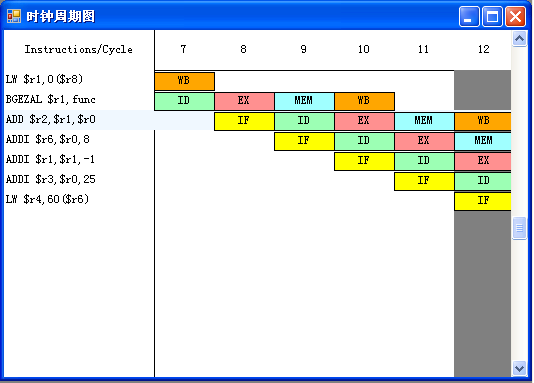（6）这时各流水寄存器中的内容为： IF/ID.IR：2361655356 IF/ID.NPC：48 ID/EX.A：0 ID/EX.B：0 ID/EX.Imm：25 ID/EX.IR： 537067545 EX/MEM.ALUo：4 EX/MEM.IR：539099135 MEM/WB.LMD：0 MEM/WB.ALUo：8 MEM/WB.IR：537264136 （7）观察和分析结构冲突对CPU性能的影响，步骤如下： • 1）加载structure_hz.s（在模拟器所在文件夹下的“样例程序”文件夹中）。 • 2）执行该程序，找出存在结构冲突的指令fadd, 对以及导致结构冲突的部件：：浮点加法器。 • 3）记录由结构冲突引起的停顿时钟周期数，计算停顿时钟周期数占总执行周期数的百分比：35/52=67.30769%。 • 4）把浮点加法器的个数改为4个。 • 5）再次重复步骤①~③的工作。 • 6）分析结构冲突对CPU性能的影响，讨论解决结构冲突的方法。 结构冲突对CPU性能的影响：当发生冲突时，流水线会出现停顿从而降低CPU的性能 解决结构冲突的方法：在流水线处理机中设置相互独立的指令寄存器和数据寄存器 （8）观察数据冲突并用定向技术来减少停顿，步骤如下： • 1）全部复位。 • 2）加载data_hz.s（在模拟器所在的文件夹下的“样例程序”文件夹中）。 • 3）关闭定向功能。这是通过“配置”->“定向”（是该项前面没有√号）来实现的。 • 4）用单步执行一个周期的方式（F7）执行该程序，同时查看时钟周期图，列出在什么时刻发生了RAW（先写后读）冲突。 答：在Cycle 4, 6, 7, 9, 10, 13, 14, 17, 18, 20, 21, 25, 26, 28, 29, 32, 33, 36, 37, 39, 40, 44, 45, 47, 48, 51, 52, 55, 56, 58, 59时，发生RAW冲突。 • 5）记录数据冲突引起的停顿时钟周期数以及程序执行的总时钟周期数，计算停顿时钟周期数占总执行周期数的百分比。 答：数据冲突引起的停顿时钟周期数为：31，程序执行的总时钟周期数为：65，停顿时钟周期数占总执行周期数的百分比为31/65=47.69231% • 6）复位CPU 。 • 7）打开定向功能。这是通过“配置”->“定向”（是该项前面有√号）来实现的。 • 8）用单步执行一个周期的方式（F7）执行该程序，同时查看时钟周期图，列出在什么时刻发生了RAW（先写后读）冲突，并与步骤3的结果进行比较。 答：在第5,9,13,17,21,25,29,33,37发生了RAW冲突，可以看到，通过定向技术，大大 减少了RAW冲突数目。 • 9）记录数据冲突引起的停顿时钟周期数以及程序执行的总时钟周期数。计算采用定向技术后的性能是原来的几倍。 答：数据冲突引起的停顿周期数为：9，程序的总时钟周期数为：43，停顿时钟周期数占总执行周期数的百分比为9/43=20.93023%，采用定向技术后的性能是原来的65/43=1.51倍。 ### 二 指令调度和延迟分支 1. 启动MIPSsim 2. 根据预备知识中关于流水线各段操作的描述，进一步理解流水线中各段的功能，掌握各流水线的含义（用鼠标双击各段，就可以看到各流水寄存器的内容） 3. 勾选配置菜单中的“流水方式”，使模拟器工作于流水方式下 4. 用指令调度技术解决流水线中结构冲突和数据冲突 a) 启动MIPSsim b) 通过“配置”菜单中的“常规配置”项把加法、乘法、除法部件的个数设置为两个，把他们的延迟时间都设置为3个时钟周期 c) 用MIPSsim的“文件”菜单中的“载入程序”来加载schedule.s（在模拟器文件夹下的“样例程序”中） d) 关闭定向功能 e) 执行所载入的程序，通过查看统计数据和时钟周期图，找出并记录程序执行过程中各种冲突发生的次数、发生冲突的指令组合，以及程序执行的总时钟周期数:33个 答：各种冲突发生的次数： RAW停顿：16，占周期总数的百分比：48.48485%，其中load停顿：6，占所有RAW停顿的百分比：37.5% 自陷停顿：1，占周期总数的百分比：3.030303% 停顿周期总数：17，占周期总数的百分比：51.51515% 发生冲突的指令组合： LW$r2,0($r1) 和 ADD$r4,$r0,$r2
ADD $r4,$r0,$r2 和 SW$r4,0($r1) SW$r4,0($r1) 和 LW$r6,4($r1) ADD$r8,$r6,$r1 和 MUL $r12,$r10,$r1 ADD$r16,$r12,$r1 和 ADD $r18,$r16,$r1 ADD$r18,$r16,$r1 和 SW $r18,16($r1)
SW $r18,16($r1) 和 LW $r20 8($r1)
MUL $r22,$r20,$r14 和 MUL$r24,$r26,$r14
f) 采用指令调度技术对程序进行指令调度，消除冲突。将调度后的程序放到afterschedule.s中
g) 载入afterschedule.s
after-schedule.s指令代码如下：
.text
main:
ADDIU $r1,$r0,A
MUL $r22,$r20,$r14 LW$r2,0($r1) MUL$r24,$r26,$r14
ADD $r4,$r0,$r2 LW$r6,4($r1) SW$r4,0($r1) ADD$r8,$r6,$r1
MUL $r12,$r10,$r1 ADD$r18,$r16,$r1
ADD $r16,$r12,$r1 SW$r18,16($r1) LW$r20,8($r1) TEQ$r0,$r0 .data A: .word 4,6,8 h) 执行该程序，观察程序在流水线中的执行情况，记录程序的总时钟周期数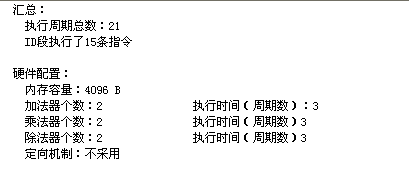指令调度后的总周期数为21。 i) 根据记录结果，比较调度前和调度后的性能。论述指令调度对于提高CPU的作用 答：调度前的执行周期为33，调度后的执行周期数为21。指令调度可以消除部分的数据冲突，通过使用指令调度提高了CPU的使用率，大大减少了指令冲突的次数，提高了CPU性能。 5. 用延迟分支减少分支指令对性能的影响 a) 启动MIPSsim b) 载入branch.asm c) 关闭延迟分支功能。这是通过在“配置”菜单中去选“延迟分支”来实现的 d) 执行该程序，观察并记录发生分支延迟的时刻，保存下其时钟周期图 答：分支延迟的时刻：Cycle 6, 9, 13, 21, 24, 28. 时钟周期图如下：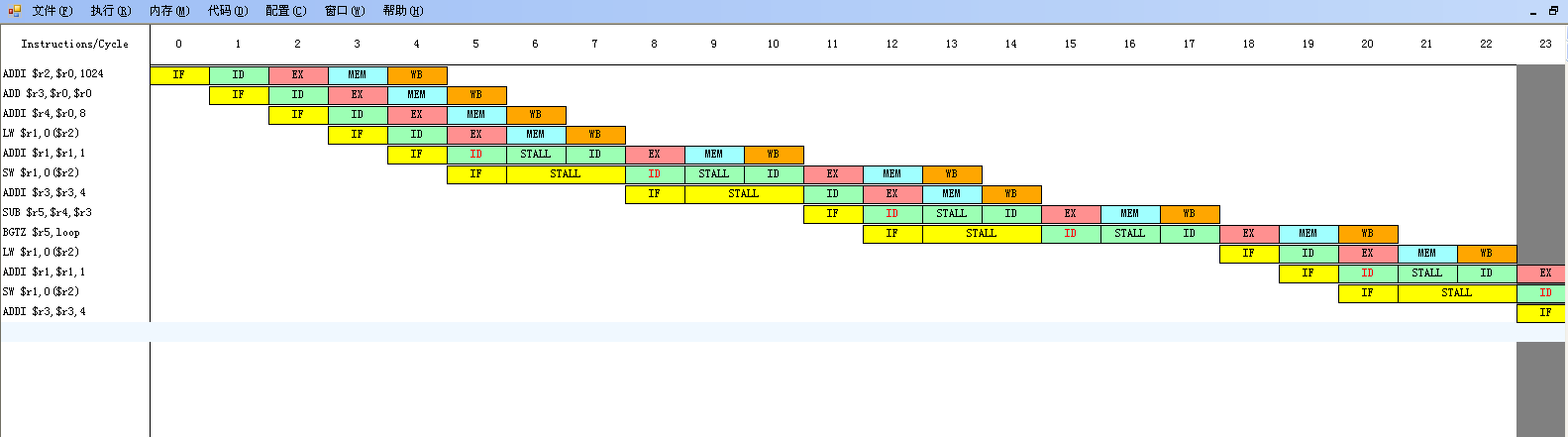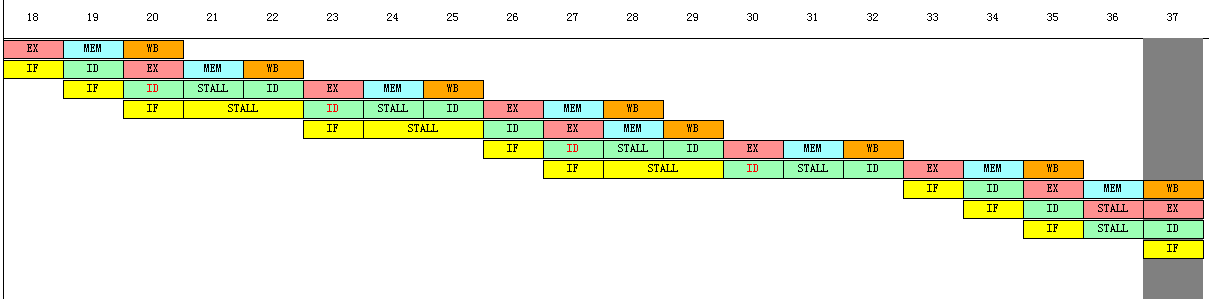e) 记录执行该程序所花的时钟周期数:38 假设延迟槽为一个，对branch.asm进行指令调度，然后存到delay-branch.s中 delay-branch.s的指令代码: .text main: ADDI$r2,$r0,1024 ADD$r3,$r0,$r0
ADDI $r4,$r0,8
loop:
LW $r1,0($r2)
ADDI $r1,$r1,1
ADDI $r3,$r3,4
SUB $r5,$r4,$r3 SW$r1,0($r2) BGTZ$r5,loop
ADD $r7,$r0,$r6 TEQ$r0,\$r0
f) 载入delayed-branch.asm
g) 打开延迟分支功能
h) 执行该程序，观察其时钟周期图，保存下其时钟周期图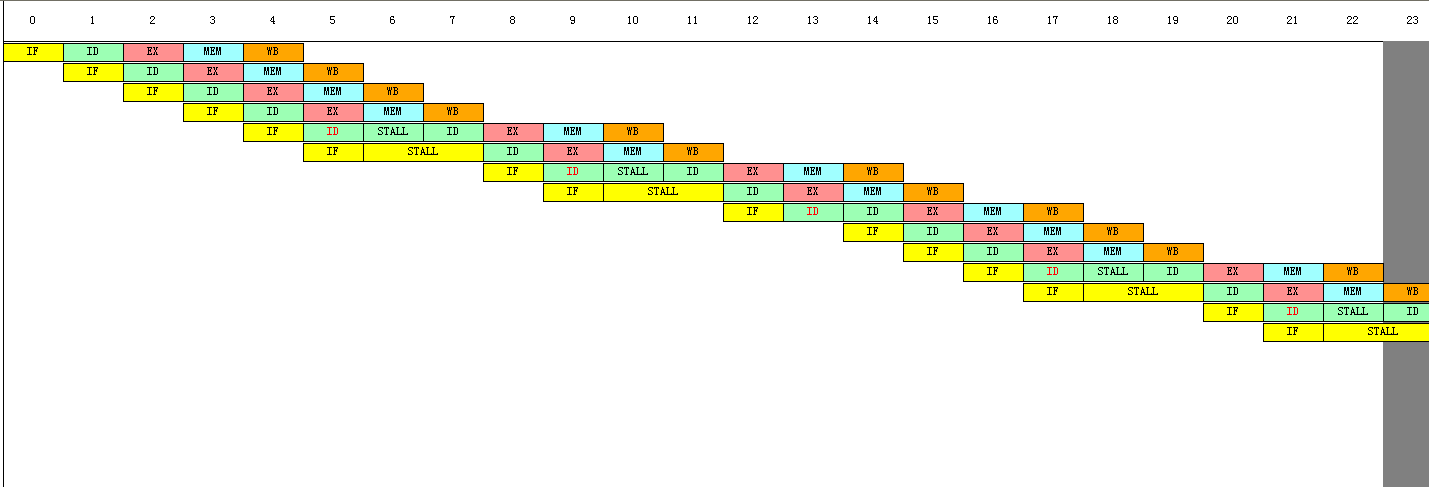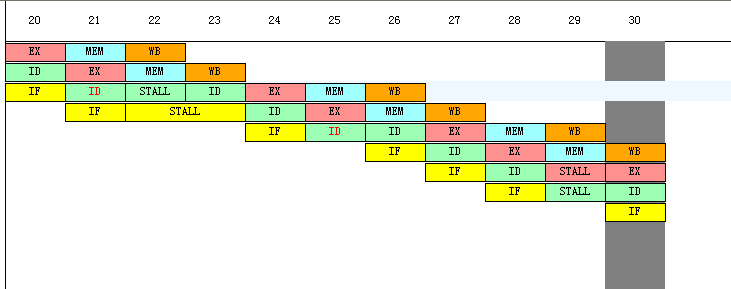i) 对比上述两种情况下的时钟周期图
j) 根据记录结果，比较没有采用延迟分支的性能和采用了延迟分支的性能。论述延迟分支对于提高CPU性能的作用
答：比较两种情况的时钟周期总数，可知：在使用延迟槽后，指令在运行到跳转指令时，不会出现延迟等待，则能够提高CPU的性能。